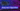# Heap Sort Algorithm

July 03, 2019This is another very useful sorting algorithm based on Heap data structure. Read more about Heap Data Structure

In this algorithn, we first prepare heap which satisfies max-heap property. Once we get out max heap ready. We get maximum element at the top. We swap this element with the last index element. And, reduce heapsize by one. Now, since we have put a newer element on top. We will just run max_heapify() algorithm on this array with new heapsize.

Finally we get the sorted array.

## Heap Sort Algorithm

• Build a max heap (using max_heapify())
• In a loop starting from last index
• swap 0-index value with current index value (which is the last index in our heap)
• reduce heap size by one. Means, largest element is done. Lets work on remaining array.
• Run max_heapify() on 0-index

See the code here:

``````public class HeapSort {
private int[] arr;
private int heapsize;

public HeapSort(int[] arr) {
this.arr = arr;
this.heapsize = this.arr.length;
}

private int getLeftChild(int index) {
return (index * 2) + 1;
}
private int getRightChild(int index) {
return (index * 2) + 2;
}

private void max_heapify(int index) {
int l = this.getLeftChild(index);
int r = this.getRightChild(index);

int indexOfLargest = index;
if (l < this.heapsize && this.arr[l] > this.arr[index]) {
indexOfLargest = l;
}

if (r < this.heapsize && this.arr[r] > this.arr[indexOfLargest]) {
indexOfLargest = r;
}

if (indexOfLargest != index) {
ArrayUtils.swap(this.arr, index, indexOfLargest);
this.max_heapify(indexOfLargest);
}
}

private void buildMaxHeap() {
for (int i=this.heapsize/2; i>=0; i--) {
this.max_heapify(i);
}
}

public void doHeapSort() {
this.buildMaxHeap();
int l = this.arr.length;
for (int i=l-1; i>0; i--){
ArrayUtils.swap(this.arr, 0, i);
this.heapsize--;

this.max_heapify(0);
}
}
}``````

## Graphical Example## Key Points

• This is an in-place algorithm.
• Uses Heap data structure
• Performance is very good. its complexity is O(n log n)

## Runtime complexity

The algorithm runs on O(n log n) in worst/average case.